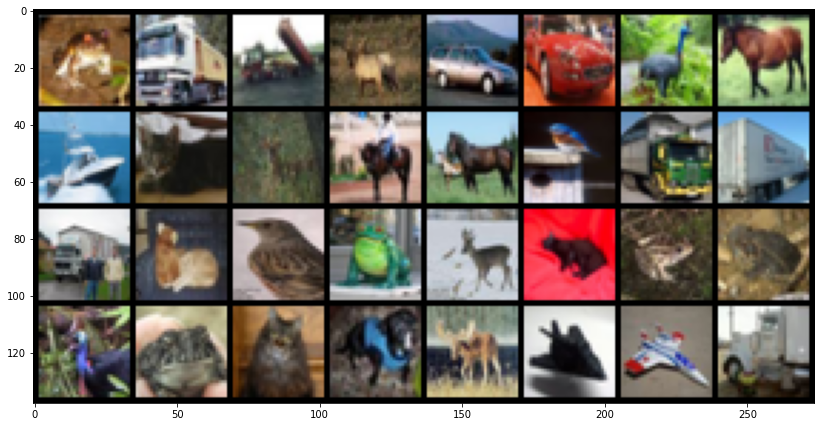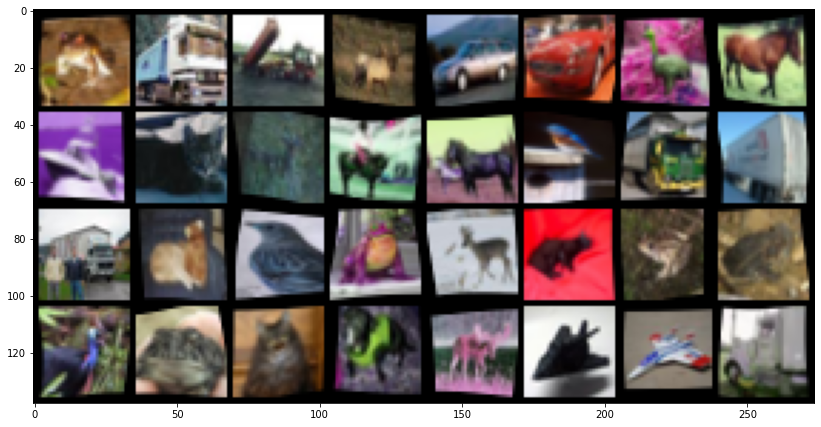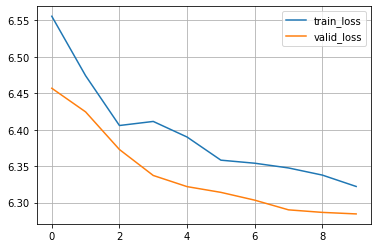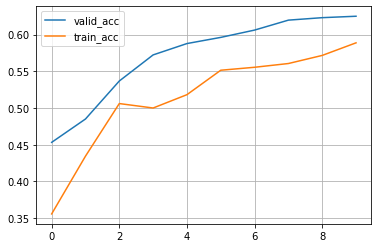Shortcuts

# GPU and batched data augmentation with Kornia and PyTorch-Lightning¶

• Author: PL/Kornia team

• Generated: 2021-09-09T15:08:26.551356

In this tutorial we will show how to combine both Kornia.org and PyTorch Lightning to perform efficient data augmentation to train a simpple model using the GPU in batch mode without additional effort.

Give us a ⭐ on Github | Check out the documentation | Join us on Slack

## Setup¶

This notebook requires some packages besides pytorch-lightning.

:

# ! pip install --quiet "torchvision" "torchmetrics>=0.3" "pandas" "matplotlib" "torchmetrics" "kornia" "pytorch-lightning" "torch>=1.6, <1.9" "pytorch-lightning>=1.3"

:

import os

import matplotlib.pyplot as plt
import numpy as np
import pandas as pd
import torch
import torch.nn as nn
import torchmetrics
import torchvision
from kornia import image_to_tensor, tensor_to_image
from kornia.augmentation import ColorJitter, RandomChannelShuffle, RandomHorizontalFlip, RandomThinPlateSpline
from pytorch_lightning import LightningModule, Trainer
from pytorch_lightning.loggers import CSVLogger
from torch import Tensor
from torch.nn import functional as F
from torchvision.datasets import CIFAR10

AVAIL_GPUS = min(1, torch.cuda.device_count())


## Define Data Augmentations module¶

Kornia.org is low level Computer Vision library that provides a dedicated module kornia.augmentation <https://kornia.readthedocs.io/en/latest/augmentation.html>__ module implementing en extensive set of data augmentation techniques for image and video.

Similar to Lightning, in Kornia it’s promoted to encapsulate functionalities inside classes for readability and efficiency purposes. In this case, we define a data augmentaton pipeline subclassing a nn.Module where the augmentation_kornia (also subclassing nn.Module) are combined with other PyTorch components such as nn.Sequential.

Checkout the different augmentation operators in Kornia docs and experiment yourself !

:

class DataAugmentation(nn.Module):
"""Module to perform data augmentation using Kornia on torch tensors."""

def __init__(self, apply_color_jitter: bool = False) -> None:
super().__init__()
self._apply_color_jitter = apply_color_jitter

self.transforms = nn.Sequential(
RandomHorizontalFlip(p=0.75),
RandomChannelShuffle(p=0.75),
RandomThinPlateSpline(p=0.75),
)

self.jitter = ColorJitter(0.5, 0.5, 0.5, 0.5)

def forward(self, x: Tensor) -> Tensor:
x_out = self.transforms(x)  # BxCxHxW
if self._apply_color_jitter:
x_out = self.jitter(x_out)
return x_out


## Define a Pre-processing module¶

In addition to the DataAugmentation modudle that will sample random parameters during the training stage, we define a Preprocess class to handle the conversion of the image type to properly work with Tensor.

For this example we use torchvision CIFAR10 which return samples of PIL.Image, however, to take all the advantages of PyTorch and Kornia we need to cast the images into tensors.

To do that we will use kornia.image_to_tensor which casts and permutes the images in the right format.

:

class Preprocess(nn.Module):
"""Module to perform pre-process using Kornia on torch tensors."""

def forward(self, x) -> Tensor:
x_tmp: np.ndarray = np.array(x)  # HxWxC
x_out: Tensor = image_to_tensor(x_tmp, keepdim=True)  # CxHxW
return x_out.float() / 255.0


## Define PyTorch Lightning model¶

The next step is to define our LightningModule to have a proper organisation of our training pipeline. This is a simple example just to show how to structure your baseline to be used as a reference, do not expect a high performance.

Notice that the Preprocess class is injected into the dataset and will be applied per sample.

The interesting part in the proposed approach happens inside the training_step where with just a single line of code we apply the data augmentation in batch and no need to worry about the device. This means that our DataAugmentation pipeline will automatically executed in the GPU.

:

class CoolSystem(LightningModule):
def __init__(self):
super().__init__()
# not the best model: expereiment yourself
self.model = torchvision.models.resnet18(pretrained=True)

self.preprocess = Preprocess()  # per sample transforms

self.transform = DataAugmentation()  # per batch augmentation_kornia

self.accuracy = torchmetrics.Accuracy()

def forward(self, x):
return F.softmax(self.model(x))

def compute_loss(self, y_hat, y):
return F.cross_entropy(y_hat, y)

def show_batch(self, win_size=(10, 10)):
def _to_vis(data):
return tensor_to_image(torchvision.utils.make_grid(data, nrow=8))

# get a batch from the training set: try with val_datlaoader :)
imgs_aug = self.transform(imgs)  # apply transforms
# use matplotlib to visualize
plt.figure(figsize=win_size)
plt.imshow(_to_vis(imgs))
plt.figure(figsize=win_size)
plt.imshow(_to_vis(imgs_aug))

def training_step(self, batch, batch_idx):
x, y = batch
x_aug = self.transform(x)  # => we perform GPU/Batched data augmentation
y_hat = self(x_aug)
loss = self.compute_loss(y_hat, y)
self.log("train_loss", loss, prog_bar=False)
self.log("train_acc", self.accuracy(y_hat, y), prog_bar=False)
return loss

def validation_step(self, batch, batch_idx):
x, y = batch
y_hat = self(x)
loss = self.compute_loss(y_hat, y)
self.log("valid_loss", loss, prog_bar=False)
self.log("valid_acc", self.accuracy(y_hat, y), prog_bar=True)

def configure_optimizers(self):
scheduler = torch.optim.lr_scheduler.CosineAnnealingLR(optimizer, self.trainer.max_epochs, 0)
return [optimizer], [scheduler]

def prepare_data(self):



## Visualize images¶

:

# init model
model = CoolSystem()

Downloading: "https://download.pytorch.org/models/resnet18-5c106cde.pth" to /home/AzDevOps_azpcontainer/.cache/torch/hub/checkpoints/resnet18-5c106cde.pth

:

model.show_batch(win_size=(14, 14))

Downloading https://www.cs.toronto.edu/~kriz/cifar-10-python.tar.gz to /__w/2/s/cifar-10-python.tar.gz

Extracting /__w/2/s/cifar-10-python.tar.gz to /__w/2/s## Run training¶

:

# Initialize a trainer
trainer = Trainer(
progress_bar_refresh_rate=20,
gpus=AVAIL_GPUS,
max_epochs=10,
logger=CSVLogger(save_dir="logs/", name="cifar10-resnet18"),
)

# Train the model ⚡
trainer.fit(model)

GPU available: True, used: True
TPU available: False, using: 0 TPU cores
IPU available: False, using: 0 IPUs

Files already downloaded and verified

LOCAL_RANK: 0 - CUDA_VISIBLE_DEVICES: [0,1]

| Name       | Type             | Params
------------------------------------------------
0 | model      | ResNet           | 11.7 M
1 | preprocess | Preprocess       | 0
2 | transform  | DataAugmentation | 0
3 | accuracy   | Accuracy         | 0
------------------------------------------------
11.7 M    Trainable params
0         Non-trainable params
11.7 M    Total params
46.758    Total estimated model params size (MB)

Files already downloaded and verified

/home/AzDevOps_azpcontainer/.local/lib/python3.9/site-packages/pytorch_lightning/trainer/data_loading.py:105: UserWarning: The dataloader, val dataloader 0, does not have many workers which may be a bottleneck. Consider increasing the value of the num_workers argument (try 12 which is the number of cpus on this machine) in the DataLoader init to improve performance.
rank_zero_warn(
/tmp/ipykernel_473/711885801.py:14: UserWarning: Implicit dimension choice for softmax has been deprecated. Change the call to include dim=X as an argument.
return F.softmax(self.model(x))

Files already downloaded and verified

/home/AzDevOps_azpcontainer/.local/lib/python3.9/site-packages/pytorch_lightning/trainer/data_loading.py:105: UserWarning: The dataloader, train dataloader, does not have many workers which may be a bottleneck. Consider increasing the value of the num_workers argument (try 12 which is the number of cpus on this machine) in the DataLoader init to improve performance.
rank_zero_warn(


### Visualize the training results¶

:

metrics = pd.read_csv(f"{trainer.logger.log_dir}/metrics.csv")

aggreg_metrics = []
agg_col = "epoch"
for i, dfg in metrics.groupby(agg_col):
agg = dict(dfg.mean())
agg[agg_col] = i
aggreg_metrics.append(agg)

df_metrics = pd.DataFrame(aggreg_metrics)
df_metrics[["train_loss", "valid_loss"]].plot(grid=True, legend=True)
df_metrics[["valid_acc", "train_acc"]].plot(grid=True, legend=True)

   train_loss  train_acc  epoch  step  valid_loss  valid_acc
0    6.817791    0.09375      0    49         NaN        NaN
1    6.817420    0.09375      0    99         NaN        NaN
2    6.719788    0.18750      0   149         NaN        NaN
3    6.708793    0.25000      0   199         NaN        NaN
4    6.622213    0.31250      0   249         NaN        NaN

:

<AxesSubplot:>## Tensorboard¶

:

# Start tensorboard.
# # %tensorboard --logdir lightning_logs/


## Congratulations - Time to Join the Community!¶

Congratulations on completing this notebook tutorial! If you enjoyed this and would like to join the Lightning movement, you can do so in the following ways!

### Star Lightning on GitHub¶

The easiest way to help our community is just by starring the GitHub repos! This helps raise awareness of the cool tools we’re building.

The best way to keep up to date on the latest advancements is to join our community! Make sure to introduce yourself and share your interests in #general channel

### Contributions !¶

The best way to contribute to our community is to become a code contributor! At any time you can go to Lightning or Bolt GitHub Issues page and filter for “good first issue”.

### Great thanks from the entire Pytorch Lightning Team for your interest !¶